Reach Us+44-1522-440391
Laser Dynamics: Weak versus Strong Harmonic-Expansion Modelling | OMICS International
Journal of Lasers, Optics & Photonics
All submissions of the EM system will be redirected to Online Manuscript Submission System. Authors are requested to submit articles directly to Online Manuscript Submission System of respective journal.

# Laser Dynamics: Weak versus Strong Harmonic-Expansion Modelling

Belkacem Meziane*

Université d’Artois, UCCS Artois, UMR CNRS 8181, Rue Jean Souvraz, SP 18, 62307, Lens Cedex, France

*Corresponding Author:
Belkacem Meziane
Université d’Artois, UCCS Artois
UMR CNRS 8181, Rue Jean Souvraz, SP 18
62307, Lens Cedex, France
Tel: +33 (0) 3 21 79 17 32
E-mail: [email protected]

Received Date: October 15, 2014; Accepted Date: November 26, 2014; Published Date: December 06, 2014

Citation: Meziane B (2014) Laser Dynamics: Weak versus Strong Harmonic-Expansion Modelling. J Laser Opt Photonics 1:110. doi:10.4172/2469-410X.1000110

Copyright: © 2014 Meziane B. This is an open-access article distributed under the terms of the Creative Commons Attribution License, which permits unrestricted use, distribution, and reproduction in any medium, provided the original author and source are credited.

Visit for more related articles at Journal of Lasers, Optics & Photonics

#### Abstract

This paper aims at to providing a self-consistent outline of new analytical prospects that deal with the laser dynamics issue, from which results that go beyond the well-known linear stability analysis are extracted. The method of investigation is rooted in weak and strong- harmonic Fourier-expansions applied to the laser equations when these operate under unstable conditions. Both routines are shown to apply equally well to the simple Lorenz-Haken model as well as to the much complex integro-differential Maxwell-Bloch equations

#### Keywords

Laser dynamics; Lorenz-Haken model; Maxwell-Bloch equations; Laser signal; Broadened laser

#### Introduction

Throughout more than three decades of research pertaining to the laser dynamics issue, the analytical prospects remained always limited to the standard Linear Stability Analysis, applied to extract the conditions under which the system becomes unstable and delivers non-steady state signals, yet being supplied with some continuous pumping mechanism [1-9]. Such analysis allows for the recognition of a second-laser threshold, beyond which the system provides no more continuous outputs. Instead of stable signals, it exhibits an atypically restless behavior in the form of small oscillations that amplify with time, ultimately transforming into some periodic or aperiodic selfpulsing solutions, depending on the system’s control-parameters values [5,9-11].

Recently, we have proposed a new method of approach based on a harmonic-expansion analysis, which consists in the application of adjusted Fourier series to the pulsing solutions [6,7]. A judicious iterative procedure allows for the extraction of the first few orders of the series. Such an approach gives new analytical information that goes well beyond the usual second laser threshold, extracted from LSA. In particular, closed form expressions that are valid at, below, and above the instability threshold are pulled-out from the simple Lorenz-Haken model as well as from the much more complex integro-differential Maxwell-Bloch equations [2,4,7]. The complexity of this second model stems from the inhomogeneous nature of the laser-line broadening, owing to the Doppler Effect associated with permanent motion of the lasing atoms, inside a gas laser, for example.

Since the unsteady solutions divide themselves into a transient part, with initially small amplitude oscillations, and a long-term one with much stronger pulsations, these distinct features naturally call for adjusted Fourier series, respectively in the form of small and strongharmonic expansions. These expansions represent the signatures of the solutions, whose first few components are shown to carry most of the fundamental analytical information, straightforwardly derived from simple algebraic manipulations. Despite the seemingly irresoluble differences between the simple Lorenz-Haken model and the complex integro-differential equations, the main analytical results are directly pulled-out from the same iterative procedure, conducted up to thirdorder in electric field amplitude, for both models.

#### Basic Laser Scheme and Theoretical Grounds

In its simplest geometry, as represented in Figure 1, a laser cavity involves three main components: 1-A pair of perfectly parallel mirrors, one of which is totally opaque to the laser wavelength, while the other, from which exits the useful part of the laser signal, ensures some partial transmittance; 2-An initially absorbing (at thermal equilibrium) medium positioned between the two mirrors; and 3-An external energy source that breaks the thermo-dynamical equilibrium and transforms the absorbing material into an amplifying-one through judicious excitation mechanisms. These mechanisms support atomic or molecular energy transfer from lower to higher levels, ultimately resulting in population inversion which, in turn, seeds and amplifies the oscillating signal inside the cavity.

Three variables and four control parameters govern light-matter interactions inside the system, namely the electric field E(t) associated to the electromagnetic signal, with a relaxation rate (inverse of the cavity lifetime), hereafter designated ĸ, whose value essentially relates to the length of the cavity and to the reflection coefficient of the output mirror; a macroscopic polarization P(t), and a population inversion D(t) that both characterize the excited medium, with respective relaxation rates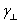and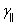. An excitation parameter, hereafter designated 2C, quantifies the strength of the external pumping source. E(t), P(t) and D(t) represent the system’s variables;ĸ,,, and 2C, its control parameters.

Basic Laser theory is founded on the well-known Lamb selfconsistent analysis. The physical grounds of such a theory are easily grabbed with the help of the following diagram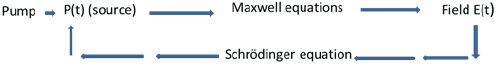Schematic representation of the Lamb self-consistent analysis indicating the basic theoretical ingredients of light-matter interactions that take place inside a Laser.

The external energy source (pump) induces oscillating dipole moments p(t) at a microscopic level, giving rise, when summed-up, to a macroscopic polarization P(t), which serves as a driving term in Maxwell’s equations that handle the propagating properties of the electromagnetic field E(t) oscillating inside the cavity. The field and polarization, in turn, interact inside the amplifying medium, energy of the form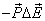. Such an energy transforms into a perturbing Hamiltonian to involve interaction, which when injected into the Schrödinger equation that handles the atomic energy transitions, yields the evolution of P(t) and D(t). Non-linear differential equations that describe light-matter interactions inside an amplifying medium are finally extracted following some valid approximations. Hereafter, we will focus on the two basic models obtained from the above pictorial elements, the single mode homogeneously broadened laser, and the in homogeneosuly broadened system.

The single-mode homogeneously-broadened “Lorenz-Haken” model

The simplest model that describes light-matter interactions inside an oscillating cavity is that of a single-mode homogeneously broadened and unidirectional ring laser. This model stems from the above Lamb self-consistent analysis, provided some fairly suitable physical estimates are taken into account. The most fundamental assessments are the Slowly Varying Envelope and the Rotating Wave Approximations. These two assumptions project laser theory into the framework of three non-linearly coupled differential equations that take the following simple normalized form [1,5,7].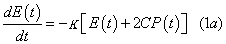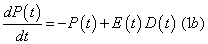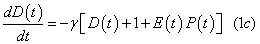Excitation parameter 2C quantifies the pumping strength with respect to its level at lasing threshold,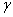and ĸ are normalized quantities with respect to the polarization relaxation ratewhile t is a dimensionless variable representing the product of time withIn most lasers, the polarization P(t) is too fast to track, with respect to the much slower variables E(t) and D(t). In such cases, an adiabatic approximation is applied to Eq. (1b). It consists in setting the derivative equal to zero, yielding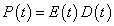to be injected in Eqs (1a) and (1c). As a result, the three-dimensional set (1) is projected into a twodimensional one, the well-known rate equations, whose dynamics is strictly limited to transient oscillations relaxing towards steady permanent state when these undergo some small external perturbation. It is an easy task to demonstrate that the rate equations are dynamically stable and always return to their state of equilibrium if perturbed.

In other cases however, and under some conditions, namely a bad cavity configuration, for which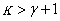and a level of pumping, which must exceed some specific value, termed as “second laser threshold”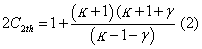the above equations become unstable. Experiencing some slight perturbation, the solutions depart from the initially stable steady state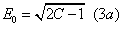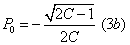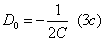obtained when all the derivatives in Eqs (1) are set equal to zero.

Typical periodic solution :When the bad cavity condition is satisfied and when the system is driven beyond the instability threshold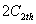the solutions of Eqs (1) move off the permanent state (3). Such a departure takes the form of small amplitude oscillations with growing amplitude during some period of time, referred to as the transient regime, until it finally settles in a long-term pulsing regime. As indicated in Figure 2, these two regimes carry distinct features; the signal first grows with small oscillations around the steady-state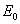before terminating with strong amplitude oscillations around zero-mean value for the electric field. It thus becomes obvious that the transient and the “permanent” states cannot be handled with the same analytical approach. The typical solution represented in Figure 2 has been numerically simulated with 2C=10, K=3, and γ = 0.1, and an initial condition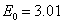, for the electric field.

Figure 2: Transient and Long-term solutions of Eqs (1), simulated at the onset of instability, with 2C=10, k=3 , and γ=0.1.

The distinctive features between the transient and the permanent parts of the solution will serve as the main guidelines for the weak and strong-harmonic expansion analyses developed in the following paragraphs.

Weak-harmonic expansion approach versus linear stability analysis: Usual analytical diagnoses, which may be found in any laser textbook [5,9], are limited to the standard Linear stability Analysis. Such a diagnosis allows for the extraction of the bad cavity condition along with an expression for the second laser threshold (Eq. (2)), under which a small perturbation grows to depart from the initial steady-state (Eqs (3)). In addition, a closed form expression that corresponds to the transient-signal frequency- fluctuation is also extracted. The standard LSA method assumes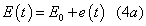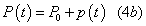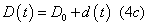where e(t), p(t) and d(t) are small quantities with respect to Eο , Pο , and Dο respectively.

When these expressions are plugged into Eqs (1), assuming the small perturbations to evolve according to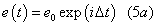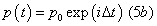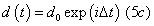one obtains a first expression for the instability threshold, as given by Eq. (2), and a second formulae that provides the angular frequency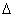at which the developing signal oscillates, at this instability threshold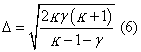Apart from expressions (2) and (6) (only valid, let us insist, at the onset of instability) LSA gives no more analytical information! The features of the transient signal or those of the long-term solution, away from the instability threshold, have always called for direct numerical simulations of Eqs (1).

The main weakness of the LSA procedure may be pointed-out from a simple glance at Figure 3, representing the transient parts of the three interacting variables. As these depictions clearly indicate, electric field, polarization and population inversion are not phase locked, whereas, through Eqs (5), LSA imposes an in-phase evolution to all three variables. Such a phase locking hypothesis is in total contradiction to the competing nature of the non-linearly coupled Eqs (1), as clearly displayed in the transient time traces of Figure 3.

Figure 3: Typical time traces in the transient regime showing clear out-ofphase evolutions between a)E(t), b)P(t), and c)D(t). d)is a Fourier transform that quantifies the frequency of the temporal signals.

In order to account for the noticeably un-locked evolution of the transient time-traces, E(t), P(t) , and D(t) are expanded in the form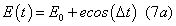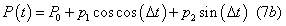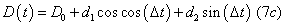Which, when inserted into Eqs (1), yield a series of 6 algebraic relations between the small amplitudes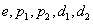and the yet unknown angular frequency Δ . After some lengthy but straightforward algebra, we obtain an expression for the angular frequency in the form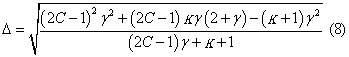Evidently revealing an explicit dependence on excitation parameter 2C, as well as on field and population-inversion relaxation rates K and γ

Indeed, when the instability threshold (Eq. (2)) is inserted in Eq. (8), one retrieves Eq. 6. This constitutes a first test of validity for the small harmonic expansion analysis. Additional confirmation must call for direct comparison between relation (8) and numerical simulations. Figure 4 represents the oscillation frequency as a function of the excitation parameter, as given by Eq. (8) (solid upper-line) and a few scattered points obtained through direct numerical simulations. The dot values were precisely quantified with the help of the Fourier transform algorithm (as in the example shown in Figure 3d).

Figure 4: Transient and long-term frequencies (solid lines) along with few numerically simulated values (dots). Both curves show excellent agreement between the closed form expressions Eq.8 (upper curve) and Eq. 12c (lower graph) with those obtained from straight numerical simulations of Eqs (1).

As expected, the validity of the analytical expression (8) extends to the whole control parameter space, be it below, at, or above the instability threshold! This constitutes a fundamentally fuller outcome as compared to the one given by linear stability analysis.

Now, let us turn to the long-term solution and search for an adapted strategy to obtain further analytical projections.

Strong-harmonic expansion analysis: Again, from a quick glance at figure 2, it transpires that the weak harmonic expansions cannot give any information on the long-term signal, since the field variable no longer oscillates around steady-state, but around the time axis, a signature of zero-average value. Typical traces of the three variables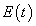,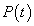,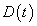,in the pulsing regime, are represented, respectively in Figures 5a, 5b, and 5c. One may directly see that the variables evolve to satisfy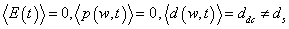Figures 5d, 5e, 5f are the Fourier spectra corresponding to each variable.

Figure 5: Long-term time traces and corresponding frequency spectra of a) the field E(t), b)polarization P(t), and c)population inversion D(t). Note the presence of odd components in the field and polarization spectra, while the population-inversion spectrum exhibits an even frequency distribution.

The times traces of Figure 5 also indicate that each variable evolves with strong amplitudes, of the same order of magnitude as the steady state values.

Accordingly, adapted expansions must take the following strongharmonic forms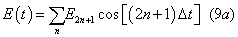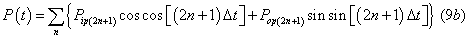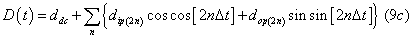n = 0, 1, 2, 3, etc.

where the subscripts ip and op stand, respectively, for in-phase and outof- phase components.

From a first sense, it is easy to understand that straightly plugging Eqs (9) into Eqs (1) would yield an inextricable infinite set of algebraic relations, from which one must find some way through. Such a way calls for the following iterative procedure.

• First step

To start with, we limit expansion (9a) to its first-order component, and write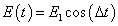(10a)

which, when inserted in Eq. (1a) carries out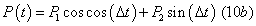P1, and P2 are then evaluated from Eq. (9b), taking into account, in a first approximation, the population-inversion sole dc-part.

The above first-order expressions for E(t) and P(t) inflict a driving term to Eq. (1c), in the form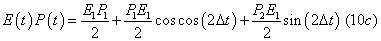imposing to Eq. (1c) a first solution of the same form. That is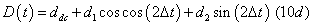Injecting back D(t) into Eq. (1c), with the use of Eq. (10c), we solve for ddc , d1 , and d2 therefore completing the first-order solutions for all three variables.

• Second step

With expressions (10a) and (10d), the driving term in Eq. (1b) expands into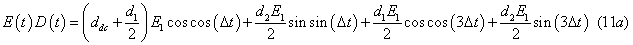Inducing the following third-order development to the polarization variablethat we insert back into Eq. (1b) to obtain a series of algebraic relationships from which we extract analytical expressions for the first and third-order field-amplitudes, along with a closed form formulae for the long-term angular-frequency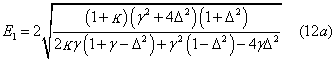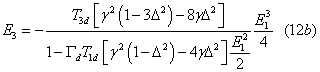and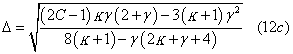Where the coefficients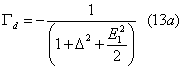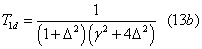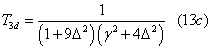have been introduced for shortening purposes. Again, an explicit dependence on excitation parameter 2C and relaxations rates K and γ clearly appears in the angular frequency formulae.

As intuitively expected, the field third-order component scales to the power three, in first- order field-amplitude. Figure 4 (lower trace, solid line) indicates a perfect match between the analytical expression (12c) and the numerically simulated long-term frequencies (dots along the curve).

It is worth to put an accent on the fact that only the above first and third order calculations are necessary in order to derive an analytical expression for the long-term frequency whose validity extends to the whole parameter space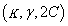.K, Its validity goes much beyond the region of period-one solutions, as those of Figure 5. It spreads over regions of the control-parameter space that comprise some regular cascading solutions, in the form of a period-doubling hierarchy, for example .

The iterative procedure may be continued towards further steps if one wishes to extract higher-order field-amplitudes. Since the third order components in field and polarization naturally induce a driving term E(t)P(t) that develops the population inversion to its fourth-order expansion, a fifth-order field-component may be extracted from Eqs (1a) and (1b), which, in turn, allows for the extraction of a seventhorder component, and so on. However, since our aim is not to obtain complete analytical solutions but rather to extract new analytical information such as the expression of the operating long-term frequency Eq. (12c), we limit our presentation to the results obtained thus far. Additional outcomes may be found in Refs [6,7,11].

We now apply the weak and strong harmonic expansion analyses to the integro-differential system, again limiting our goal to the derivation of analytical formulas that handle the transient and permanent pulsing states, in terms of oscillation frequencies.

The integro-differential « Maxwell-Bloch » system

This system is derived along the same lines of thoughts as the simpler Lorenz-Haken model, following the lamb self-consistent analysis. It describes light-matter interactions inside an in homogeneously broadened medium placed inside a judiciously arranged ring-cavity. For example, inside a gaz laser where the motion of the interacting particles results in a Doppler-shift hereafter denoted w, of the emitted radiation with respect to those at rest. A Gaussian distribution g(w), integrated over the amplifying medium, takes into account such an effect [2,4,7].

In normalized form, the integro-differential equations write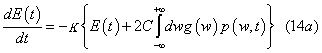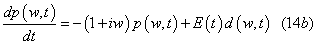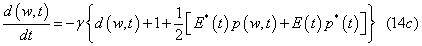The control parameters K,γ and 2C bear the same significations as those of the Lorenz-Haken model.

Despite the integral over the inhomogeneous profile in Eq. (14a), which seems to complicate the problem of light-matter interactions, the dynamical solutions of Eqs (14) bear some amazing resemblance with those of the simpler Lorenz-Haken equations. A typical example is represented in Figure 6, at the transition region from the transient to the “permanent” solution for the field (Figure 6a), and the center-line components for the polarization (Figure 6b) and population inversion (Figure 6c).

Figure 6: Time traces at the transition region from transient to permanent period-one solution of a)field E(t); -center-tuned b)polarization and c) population inversion-components obtained at the onset of instability, with k=4, and γ=0.1.

Just as in the case of the Lorenz-Haken system, the transient oscillations take place around the steady-state values for each variable, while the long term solution fluctuates around zero-mean values for the field and polarization, and around a dc value, distinct from the corresponding steady-state, for the population inversion. As a consequence, it becomes obvious that the weak and strong harmonic expansions analyses do apply in this case, yet the algebra becomes even more tedious, since each atomic packet situated at w away from linecenter participates to the dynamics, through the integral in Eq. (14a), and must be taken into account.

Weak-harmonic expansion analysis of the transient regime: The transient time traces of Figure 6 indicate small fluctuations taking place around steady state values with clear unlocked progressions of each variable with respect to the others. Thus, to first order, we may write, in the case of real fields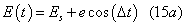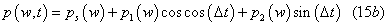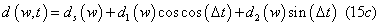When these expansions are inserted into Eqs (14), we are led to a series of algebraic relations between the small amplitudes e,p1,p2,d1,d2., that contain the yet unknown angular frequency Δ . After some lengthy but straightforward algebraic manipulations, we obtain the following two relations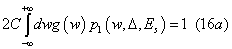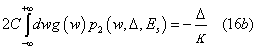the ratio of which yields an expression that encloses one unknown only, that is Δ , for fixed control parameter κ,γ and excitation level ES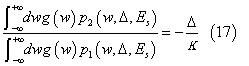The excitation parameter 2C is related to the steady-state intensity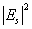through the following state equation (obtained when the derivatives in Eqs (14) are set equal to zero)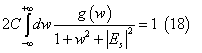Scanning over 2C thus amounts to varying ES

Equation (17) can only be solved graphically by representing its right and left-hand sides on the same diagram, for given excitation level and control parameters γ and K. An example of such a solution is represented in Figure 7.

In order to draw the characteristic curve that describes the evolution of the transient pulsation with respect to excitation level, one must scan over ES and compute the corresponding Δ value, as done in Figure 7. In so doing, one obtains a solid curve associated to Eq. (17), in which ES is the variable and Δ the function. The details of the resultant graph are represented in Figure 8. It is worth mentioning that up to twice the threshold Es=1.5, 2C=2 the frequencies of the numerical simulations exactly match those of the closed form expression (17). For higher excitations, indeed, higher order terms, neglected in this approach, participate to the transient dynamics, and the first order expression deviates from the actual frequencies of the transient regime.

Figure 7: Graphical solution of Eq. (17) giving the pulsation frequency of the transient oscillations of Figure 6. In this example, the solution reads Δ≅ 0.14.

Figure 8: Pulsation versus excitation level, corresponding to Eq. (17) (solid line) obtained with κ=4, γ=0.1. The dots were computed through direct numerical simulations of the integro-differential equations (14) along with a Fast Fourier Transform algorithm.

Strong-harmonic expansion analysis of the pulsing regime: According to the long-term time traces of figure 6, the pulsing regime carries the same properties as those of the Lorenz-Haken model, i.e..Accordingly, an intrinsic expansion of the electric field consists in a Fourier series of the form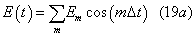While the medium’s variables follow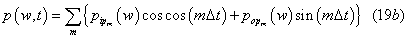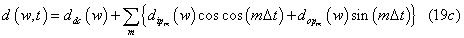Just as in the case of the Lorenz-Haken model, the third-order expansions are all we need in order to extract the necessary long-term pulsation. Careful algebraic handlings yield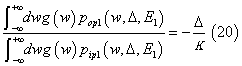n which only the value of Δ is unknown, since the first order fieldamplitude E1 is formerly determined with the first-order expansion.

At first sight, this last formula seems identical to Eq. (17). However, comparison between expansions (15b) and (19b) tells us that while p1 and p2 are small quantities with respect to ps, the values of p0p1, and pip1 are of the same order of magnitude as ps. Indeed, the obtained expressions for the in-phase and out-of phase polarization components are quite distinctive in both cases.

Equation (20) must also be solved graphically, for fixed values of the control parameters and excitation level. An example of such a solution is given in Figure 9a, while Figure 9b represents the graphical solution of the transient frequency, obtained with the same parameter values. Quick comparison between the two values reveals that the transient pulsation is almost 3 times higher than the pulsing one, as intuitively expected from the fact that the transient frequency calls for first-order developments only, while the pulsing solution requires third-order expansions.

Figure 9: Graphical solution giving a) the long-term pulsing frequency and b) the transient oscillation frequency, obtained with the same parameter values. Note that the Δ value in b) is almost 3 times higher than in a). Such a scaling conforms to the representations of Figure 6a.

Needless to emphasize on the fact that representing the left and right hand side of Eq. (20) in order to solve for Δ , as done in Figure 9b, gives the same result as when representing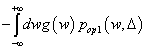on one hand, and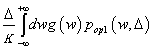on the other, as done in Figure 9a.

Despite the much more complicated algebra involved in the integro-differential “Maxwell-Bloch” equations, as compared to the Lorenz-Haken model, closed form expressions for both the transient and the long-term regimes have been derived. For each set of parameter values, we have found a fairly good match between the numerically simulated pulsations and those given by the closed form expressions, an indication of the strength of the weak and strong harmonic-expansion analyses.

Let us now turn to some instinctual and easy-to-apprehend analogies with the characteristics of a well-known academic example, the forced mass-spring system.

Simple physics versus complicated algebra: connection to resonance

Let us recall and consider the academic example of the forced harmonic oscillator; that of a mass-spring system represented in Figure 10.

Figure 10: Resonance phenomenon in the externally driven simple harmonic oscillator that occurs in the form of high-amplitude response when the frequency of an external force approaches that of the system’s intrinsic frequency ω0.

When some varying external excitation mechanism of the form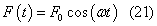is applied to the system, the movement of the mass along some x axis about its equilibrium position will take place according to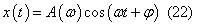The mass undergoes a displacement with frequency-dependent amplitude that gives birth to the well-known phenomenon of resonance,which occurs when the external angular frequency approaches the Eigen-pulsation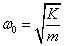of the mass-spring system (Figure 10).

In the course of our investigations, we have found that the strongharmonic expansion routine comprises additional and out-of-firstsight properties. The third order relationships simplify to end up with a closed form frequency relation that shows no dependence on excitation level, but solely depends on the characteristic parameters of the system K and γ . The obtained expression writes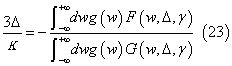Implying one single solution Δ for fixed control parameters κ and γ .

Expectedly, a characteristic frequency may also be derived from the Lorenz-Haken model with the help of the third-order fieldcomponents. It can also be derived directly from Eq. (23). Setting w=0, in Eq. (23), which amounts to selecting the center component of the profile, with the result of projecting the integro-differential equations into the three-dimensional Lorenz-Haken model, we are left with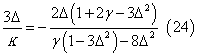which takes the simpler closed form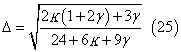Just as the resonant frequency of a spring-mass oscillator is exclusively related to the mass and the spring coefficient, the Laser intrinsic frequencies merely relate to the field and population inversion relaxation rates (both scaled to the polarization decay rate). As a consequence, the unstable solutions of both the Lorenz-Haken and the inhomogeneously broadened systems may be attributed to some resonant phenomenon which occurs when the system is excited with an external mechanism, strong enough (beyond the instability threshold) to drive the system close to its Eigen-frequency, thus giving rise to the phenomenon of resonance, which takes the form of high amplitude output pulses, such as those of the long-term time traces depicted in Figures 2, 5 and 6.

#### Conclusion

The purpose of this paper was to outline, through a self-consistent presentation, a new strategy of approach and show how analytical information that go well beyond the usual linear stability analysis can be extracted from the non-linear differential equations that govern light-matter interactions inside a bad-cavity configured laser. Closed form expressions were derived to give exact quantifications of both the transient and the long-term pulsations that characterize the laser behavior when the system is driven into its state of turbulence. Our methodology is rooted in the application of a weak and a strongharmonic expansion analyses, shown to be adapted respectively to the transient, with small oscillations, and “permanent”, with strong selfpulsing, parts of the solutions. Both methods reveal to apply equally well to the simple Lorenz-Haken model and to the more complex integro-differential Maxwell-Bloch equations. In addition, intrinsic Eigen-frequencies were shown to characterize both models. These proper frequencies bear the same significance as that of an externally driven harmonic oscillator, known to give rise to the phenomenon of resonance. The self-pulsing solutions of the laser equations may thus be viewed as the manifestation of such a resonance property, which takes place when the external pumping mechanism is raised beyond some critical level, the so-called instability threshold.

#### References

Select your language of interest to view the total content in your interested language

### Article Usage

• Total views: 12196
• [From(publication date):
December-2014 - Sep 18, 2019]
• Breakdown by view type
• HTML page views : 8410

## Post your commentCan't read the image? click here to refresh
###### Peer Reviewed Journals

Make the best use of Scientific Research and information from our 700 + peer reviewed, Open Access Journals

International Conferences 2019-20

Meet Inspiring Speakers and Experts at our 3000+ Global Annual Meetings

Top